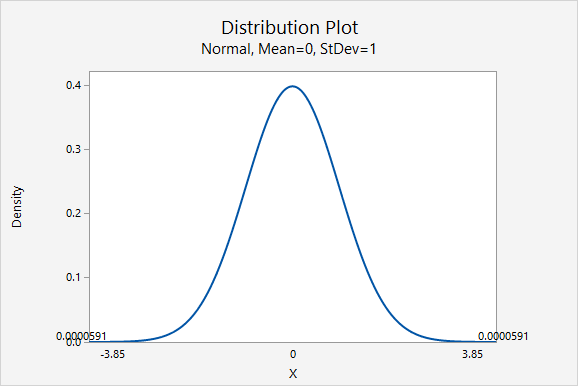# 9.1.2.1.1 – Example: Ice Cream

9.1.2.1.1 – Example: Ice Cream

## Example: Ice Cream

The Creamery wants to compare adults and children in terms of preference for eating their ice cream out of a cone. They take a representative sample of 500 customers (240 adults and 260 children) and ask if they prefer cones over bowls. They found that 124 adults preferred cones and 90 children preferred cones.

1. Check any necessary assumptions and write hypotheses.

$$H_0: p_a - p_c = 0$$

$$H_a:p_a - p_c \ne 0$$

Check assumptions:

$$n_a \hat{p}_a = 124$$

$$n_a (1-\widehat p_a) = 240 - 124 = 116$$

$$n_c \widehat p_c = 90$$

$$n_c (1-\widehat p_c) = 260-90 = 170$$

These counts are all at least 10 so we can use the normal approximation method.

2. Calculate an appropriate test statistic.
Pooled Estimate of $$p$$
$$\widehat{p}=\dfrac{\widehat{p}_1n_1+\widehat{p}_2n_2}{n_1+n_2}$$

$$\widehat{p}=\dfrac{124+90}{240+260}=\dfrac{214}{500}=0.428$$

Standard Error of $$\hat{p}$$
$$SE_{0}={\sqrt{\frac{\widehat{p} (1-\widehat{p})}{n_1}+\frac{\widehat{p}(1-\widehat{p})}{n_2}}}=\sqrt{\widehat{p}(1-\widehat{p})\left ( \frac{1}{n_1}+\frac{1}{n_2} \right )}$$

$$SE_{0}=\sqrt{0.428(1-0.428)\left ( \dfrac{1}{240}+\dfrac{1}{260} \right )}=0.04429$$

Test Statistic for Two Independent Proportions
$$z=\dfrac{\widehat{p}_1-\widehat{p}_2}{SE_0}$$

$$z=\dfrac{\dfrac{124}{240}-\dfrac{90}{260}}{0.04429}=3.850$$

3. Determine the p-value associated with the test statistic.

This is a two-tailed test. Our p-value will be the area of the $$z$$ distribution more extreme than $$3.850$$.$$p = 0.0000591 \times 2 = 0.0001182$$

4. Decide between the null and alternative hypotheses.

$$p \le 0.05$$

Reject the null hypothesis

5. State a "real world" conclusion.

There is evidence that, in the population of Creamery customers, the proportion of adults who prefer cones is different from the proportion of children who prefer cones in the population.

  Link ↥ Has Tooltip/Popover Toggleable Visibility